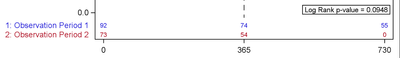## How to stop xaxistable auto numbering my formatted labels

I cannot figure out how to get the labels to stop auto numbering.  I am using 9.4 sgplot to make my Kaplan-Meier graphs, but it keeps auto numbering the xaxistable labels.  I would like to not have the 1: and 2: to the left of the labels (see below).  The variable is numeric, but formatted.  If I remove the format I get the original values without the numbering.  If I change it to a text variable, the auto numbering comes back, so don't think it has to do with it being formatted.

Code:

proc sgplot data=SurvivalPlotData noautolegend;
styleattrs datalinepatterns=(solid solid) datacolors=(blue red);
step x=time y=survival / group=stratum name='s' grouporder=ascending;
scatter x=time y=censored / markerattrs=(symbol=plus) name='c' grouporder=ascending;
scatter x=time y=censored / markerattrs=(symbol=plus) GROUP=stratum grouporder=ascending;
xaxistable atrisk / x=tatrisk location=inside class=stratum colorgroup=stratum ;
Yaxis label="% Survival" values=(0 to 1 By .2) ;
Xaxis LABEL="Days After Transplant" values=(0 to 730 By 365) ;
INSET "Log Rank p-value = 0.0948" / BORDER TEXTATTRS = (SIZE=8 COLOR=black)
position=bottomright;
refline 365 / axis=x lineattrs=(thickness=1 color=black pattern=dash);
run;1 ACCEPTED SOLUTION

Accepted SolutionsKsharp
Super User

## Re: How to stop xaxistable auto numbering my formatted labels

Open your "SurvivalPlotData" dataset and see if there is "the auto numbering " in variable "stratum" ?
2 REPLIES 2Ksharp
Super User

## Re: How to stop xaxistable auto numbering my formatted labels

Open your "SurvivalPlotData" dataset and see if there is "the auto numbering " in variable "stratum" ?

## Re: How to stop xaxistable auto numbering my formatted labels

Thank you.  The problems was the ODS from the Proc Lifetest adding the numbering, not the graphing.

Discussion stats
• 2 replies
• 153 views
• 1 like
• 2 in conversation